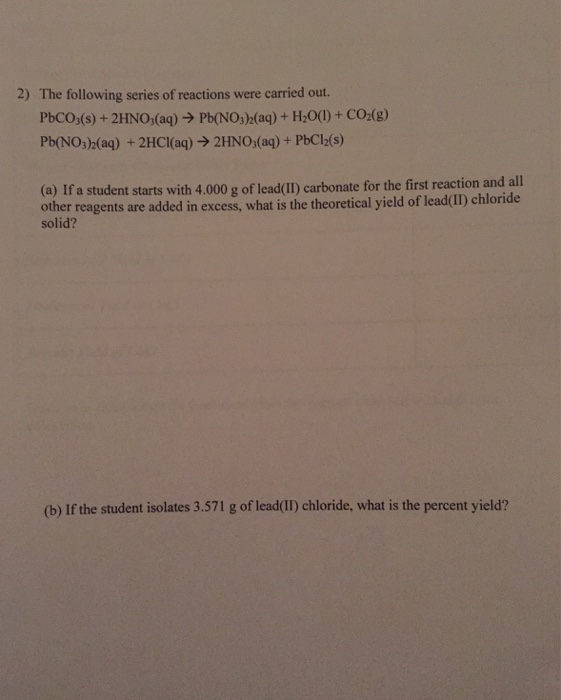# The following series of reactions were carried out. PbCO_3(s) + 2HNO_3(aq) rightarrow Pb(NO_3)_2(aq) + H_2O(l) +...

###### Question:The following series of reactions were carried out. PbCO_3(s) + 2HNO_3(aq) rightarrow Pb(NO_3)_2(aq) + H_2O(l) + CO_2(g) Pb(NO_3)_2(aq) + 2HCl(aq) rightarrow 2HNO_3(aq) + PbCl_2(s) If a student starts with 4.000 g of lead(II) carbonate for the first reaction and all other reagents are added in excess, what is the theoretical yield of lead(II) chloride solid? If the student isolates 3.571 g of lead(II) chloride, what is the percent yield?

#### Similar Solved Questions

##### 1. Use the Laplace transform to solve the following BVP au au əx2 = ət, x...
1. Use the Laplace transform to solve the following BVP au au əx2 = ət, x > 0,t > 0 u(0, 1) = tuo, lim u(x, t) = u1, t> 0, u(x,0) = U1, x > 0....
##### Problem 3) Determine the maximum shear stress in a rectangular beam 6 in. wide and 8...
Problem 3) Determine the maximum shear stress in a rectangular beam 6 in. wide and 8 in. deep produced by a shear force of 38,400 lb....